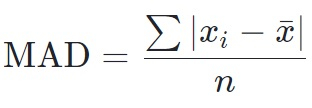# Program for Mean Absolute Deviation in C++

Given with an array of natural numbers and the task is to calculate the mean absolute deviation and for that we must require the knowledge of mean, variance and standard deviation.

There are steps that need to be followed for calculating the mean absolute deviation

• Calculate the mean

• Calculate absolute deviation

• Add all the calculated deviations

• Apply the formulaInput

arr[] = { 34,21,56,76,45,11}

Output

mean absolute deviation is : 18.5

Input

arr[] = {10, 15, 15, 17, 18, 21}

Output

mean absolute mean absolute deviation is : 2.66

## used in the given program is as follows

• Input the elements of an array

• Calculate the mean of an array

• Calculate deviation using formula Sum = Sum + abs(arr[i] - Mean(arr, n))

• calculate mean absolute deviation by dividing the total deviation with total number of elements in an array

(abs(arr – mean) + abs(arr – mean) + . . + abs(arr[n-1] – mean) / n

## Algorithm

Start
Step 1→ declare function to calculate mean
float mean(float arr[], int size)
declare float sum = 0
Loop For int i = 0 and i < size and i++
Set sum = sum + arr[i]
End
return sum / size
Step 2→ Declare function to calculate deviation
float deviation(float arr[], int size)
declare float sum = 0
Loop For int i = 0 and i < size and i++
Set sum = sum + abs(arr[i] - mean(arr, size))
End
return sum / size
Step 3→ In main()
Declare float arr[] = { 34,21,56,76,45,11}
Declare int size = sizeof(arr) / sizeof(arr)
Call deviation(arr, size)
Stop

## Example

Live Demo

#include <bits/stdc++.h>
using namespace std;
//calculate mean using mean function
float mean(float arr[], int size){
float sum = 0;
for (int i = 0; i < size; i++)
sum = sum + arr[i];
return sum / size;
}
//calculate mean deviation
float deviation(float arr[], int size){
float sum = 0;
for (int i = 0; i < size; i++)
sum = sum + abs(arr[i] - mean(arr, size));
return sum / size;
}
int main(){
float arr[] = { 34,21,56,76,45,11};
int size = sizeof(arr) / sizeof(arr);
cout<<"mean absolute deviation is : "<<deviation(arr, size);
return 0;
}

## Output

If run the above code it will generate the following output −

mean absolute deviation is : 18.5